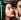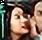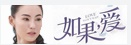• 基于非稀疏字典处理实现对低分辨率图像进行超分辨率处理
• 深度图像超分辨率重建MATLAB和C语言代码.深度图像超分辨率重建MATLAB和C语言代码.ee
• 图像超分辨率重建（ 适合初学超分辨率重建使用 图像超分辨率 SR工具箱）
• 的单幅图像超分辨率，以实现良好的 SR 性能和低失真。 您可以从以下位置查看论文 百科全书 @InProceedings{Liu2019hbpn, author = {Liu, Zhi-Song and Wang, Li-Wen and Li, Chu-Tak and Siu, Wan-Chi}, title = {...
• 基于MATLAB超分辨率重建算法，帮助学习超分辨率重建，亲测在MATLAB 2009a版本上运行无报错。超分辨率重建
• 基于MATLAB图像超分辨率重建程序，使用的是训练后的SRCNN方法，自带训练库。 (降积分)充字数........
• 图像超分辨重建/基于Matlab的多图像超分辨率重建算法程序源码 1、超分辨率重建代码 MATLAB +c 2、插值法图像超分辨率重建 matlab代码 3、分辨率图像重建matlab源码 4、超分辨率重建的matlab代码 （图像仿射变换的...

图像超分辨重建/基于Matlab的多图像超分辨率重建算法程序源码
1、超分辨率重建代码 MATLAB +c
2、插值法图像超分辨率重建 matlab代码
3、超分辨率图像重建matlab源码
4、超分辨率重建的matlab代码 （图像仿射变换的超分辨率经典程序 包括图像的配准，重建等）
5、图像超分辨重建matlab代码
6、利用POCS实现图像超分辨率重建的matlab代码
7、深层卷积神经网络实现超分辨重建 文档
8、基于正则化的图像超分辨重建matlab代码（基于正则化的图像超分辨重建与处理，用PSNR值确定重建效果）
9、基于Matlab的多图像超分辨率重建算法
10、基于MATLAB的超分辨率重建算法
11、凸集投影法（POCS）超分辨重建算法MATLAB实现（一个单一的pocs函数，使用方便）
12、图像超分辨重建MATLAB源代码（迭代步长自适应）
13、pocs超分辨率重建 程序员帮帮展开全文matlab 算法 超分辨率重建
• 利用POCS实现图像超分辨率重建matlab代码
• 图像超分辨率的实现主要就是将具有相似而又不同却又互相补充信息的配准影像融到一起，得到非均匀采样的较高分辨率数据，复原需要亚像素精度的运动矢量场，然而它们之间的运动模型估计精确与否直接影响到重建的效果...

【实例简介】

多图像超分辨率的实现主要就是将具有相似而又不同却又互相补充信息的配准影像融到一起，得到非均匀采样的较高分辨率数据，复原需要亚像素精度的运动矢量场，然而它们之间的运动模型估计精确与否直接影响到重建的效果，因此影像配准和运动模型的估计精度是高分辨率图像重建的关键。由于实际中不同时刻获得的影像数据间存在较大的变形、缩放、旋转和平移，因此必须对其进行配准，在此基础上进行运动模型估计。然后通过频率域或空间域的重建处理，生成均匀采样的超分辨率数据

【实例截图】

【核心代码】

superresolution_v_2.0

└── superresolution_v_2.0

├── __MACOSX

│   └── superresolution_v_2.0

│   └── application

│   └── html

└── superresolution_v_2.0

├── application

│   ├── applicability.m

│   ├── c2p.m

│   ├── Contents.m

│   ├── create_images.m

│   ├── estimate_motion.m

│   ├── estimate_rotation.m

│   ├── estimate_shift.m

│   ├── generatePSF.m

│   ├── generation.fig

│   ├── generation.m

│   ├── g_im_LR_1.tif

│   ├── g_im_LR_2.tif

│   ├── g_im_LR_3.tif

│   ├── g_im_LR_4.tif

│   ├── gpl

│   ├── html

│   │   └── SR_documentation.html

│   ├── interpolation.m

│   ├── iteratedbackprojection.m

│   ├── keren.m

│   ├── keren_shift.m

│   ├── logo_epfl_small.tif

│   ├── logo_warning.tif

│   ├── lowpass.m

│   ├── lucchese.m

│   ├── marcel.m

│   ├── marcel_shift.m

│   ├── n_conv.m

│   ├── n_convolution.m

│   ├── papoulisgerchberg.m

│   ├── pocs.m

│   ├── robustnorm2.m

│   ├── robustSR.m

│   ├── shift.m

│   ├── SR_documentation.m

│   ├── superresolution.fig

│   ├── superresolution.m

│   ├── wind_LR_1.tif

│   ├── wind_LR_2.tif

│   ├── wind_LR_3.tif

│   └── wind_LR_4.tif

8 directories, 43 files

展开全文• 插值法图像超分辨率重建matlab代码，可以交流学习一下超分辨率
• 超分辨率图像重建MATLAB代码或者软件专业及业余两方面的人们都是比较喜欢Photo Shop(简称PS)这个图像处理软件，这是世界知名的软件。我以本软件为例来说步骤。① 用PS打开你要处理的图片。② 执行“图像”菜单下...

求超分辨率图像重建的MATLAB代码或者软件

专业及业余两方面的人们都是比较喜欢Photo Shop(简称PS)这个图像处理软件，这是世界知名的软件。

我以本软件为例来说步骤。

① 用PS打开你要处理的图片。

② 执行“图像”菜单下的“图像大小”命令，从而打开“图像大小”对话框。

③在“图像大小”对话框中的下部有个“约束比例”，前面应该保持打勾，这可保持图像不变形。

④设定分辨率。

在“图像大小”对话框的下部有个分辨率输入框，后面的单位保持为“像素/英寸”(也就是dpi )，单位前的数值改为350 。

⑤设定宽度和高度中的一个像素数(441或者358)。

仍然在“图像大小”对话框中，上半部分有个“像素大小”区域，指定宽度或者高度的像素数为要求的大小(因为宽高比问题，不用同时指定两个，指定其中一个后，另一个自动变。

应该先指定哪个呢？应该遵照下面的原则：先试着指定其中一个值，因为约束比例，所以另一个值会自动改变，如果自动改为的数值超过所要求的数值，就是符合要求的，否则就换为先指定另一个值)。

⑥单击“确定”，从而关闭“图像大小”对话框。

⑦ 裁切超过要求的宽度或高度。

执行“图像”菜单下的“画布大小”命令，在对话框中将超过要求的宽度或高度设定为要求的值，而另外的符合要求的一方面(高度或宽度)不变。

单击“确定”，“画布大小”对话框关闭。

⑧ 保存图像，设定压缩比，使存储容量符合要求。

执行“文件”菜单下的“存储为”命令，在“存储为”对话框中的“格式”下拉框中，选择“JPEG(.....”这一项，并指定保存位置和文件名。

单击“保存”，随后出现“JPEG选项”对话框，改变“品质”的数值，从而调整图像存储的压缩程度，这会导致图像存储的容量，可以通过多次存储试验并查看文件的大小，得到14-20kb之间大小的文件。

(如果文件还大，可以使用“文件”菜单下的“存储为Web所用格式”命令)。

虽然麻烦，但是这是保证图像不变形的必要步骤。

要是你的原始图像宽高比与所要求的441*358一致，你就不用“⑦”这个步骤了。

后面给个“图像大小”对话框的图片，作为参考，里面数值自己设定。

有关提高图像分辨率带来的影响问题：

一般噪点是在像素损失的情况下才出现，怎么可能是提高的时出现呢？你是变大图像尺寸而已吧？你面积增大，单位面积的像素自然是减少，这样才空档出来就是噪点了，你可能搞错了，是放大尺寸，而不是提高像素，即成的图像是没有办法用一盘软件可以提高分辨率的，尤其是JPG图像。

你认为呢？

展开全文• 图像超分辨率,图像超分辨率重建,matlab源码
• matlab图像超分辨处理与重建的代码，基于matlab开发，界面操作的
• 传统的分辨重建算法往往采用梯度下降法进行求解，迭代时步长往往通过经验确定。而且不同的图像的最优步长往往不相同。步长过大会导致发散，步长过小会导致收敛缓慢。本算法基于对正则化分辨重建算法实现的基础上...
• 这个和前面差不多，先外框架：% 金字塔式网络实现的快速准确的超分辨率 clear all; img_filename = '测试2.jpg'; %% 参数 scale = 4; % SR 采样比例 可以是 2、4 或 8 倍 %% 设置路径 addpath(genpath('utils'));...

这个和前面差不多，

先外框架：

%        金字塔式网络实现的快速准确的超分辨率
clear all;

img_filename = '测试2.jpg';

%% 参数
scale  = 4; % SR 采样比例 可以是 2、4 或 8 倍

%% 设置路径
vl_setupnn;

%% 加载预训练模型
model_filename = fullfile('pretrained_models', sprintf('LapSRN_x%d.mat', scale));

fprintf('载入 %s\n', model_filename);
net=net.net;

%% 加载 LR 图像
fprintf('载入 %s\n', img_filename);
im_l=rgb2ycbcr(im_l);
img_LR = single(im_l(:,:,1)) /255;%;

%% 应用 LapSRN
fprintf('应用 LapSRN for %dx SR\n', scale);

%返回 2、4 和 8 倍重建（根据选中网络而定）
[im_HR1,im_HR2,im_HR3] = myLapSRN(net, img_LR);

%% 显示结果

im_H = imresize(im_l,2,'bicubic');
im_H=single(im_H);
im_H(:,:,1)= im_HR1*255;
im_H=ycbcr2rgb(uint8(im_H));
figure, imshow(im_H);title(sprintf('由  LapSRN %d 倍重建彩色', 2));
imwrite(im_H, ['LapSRN 2倍重建.jpg']);

if scale>2
im_H = imresize(im_l,4,'bicubic');
im_H=single(im_H);
im_H(:,:,1)= im_HR2*255;
im_H=ycbcr2rgb(uint8(im_H));
figure, imshow(im_H);title(sprintf('由  LapSRN %d 倍重建彩色', 4));
imwrite(im_H, ['LapSRN 4倍重建.jpg']);
end
if scale==8
im_H = imresize(im_l,8,'bicubic');
im_H=single(im_H);
im_H(:,:,1)= im_HR3*255;
im_H=ycbcr2rgb(uint8(im_H));
figure, imshow(im_H);title(sprintf('由  LapSRN %d 倍重建彩色', 8));
imwrite(im_H, ['LapSRN 8倍重建.jpg']);
end

核心函数：

% LapSRN 超分辨率重建 - 各层代码
function [hR1,hR2,hR3] = myLapSRN(net, imlow)

hR1=[];hR2=[];hR3=[];

%总层数
l=length(net.layers);

j=0;%卷积层计数，可以去掉

upsample_num=0;% 图像放大计数
residual_num=0;% 残差图计数

%循环各层直至结束
for i=1:l %

disp(['第 ',num2str(i),' 层']);
%      disp(net.layers(i).name);
%     disp(net.layers(i).type);
%     disp(net.layers(i).inputs);
%     disp(net.layers(i).outputs);
%     disp(net.layers(i).params);
%     disp(net.layers(i).block);
if strTong(net.layers(i).type,'dagnn.Conv')

j=j+1
disp('卷积层');

%得到权重（也就是卷积核）和偏移
disp('参数是：');
disp(net.layers(i).params);
fft=net.layers(i).params{1};
for k=1:length(net.params)
if strTong(net.params(k).name,fft)
weight=net.params(k).value;
break;
end
end
if net.layers(i).block.hasBias==false
bias=[]; % 无偏移
else
fft=net.layers(i).params{2};
for k=1:length(net.params)
if strTong(net.params(k).name,fft)
bias=net.params(k).value;
break;
end
end
end
%得到输入
disp('输入是：');
disp(net.layers(i).inputs);
disp('输出是：');
disp(net.layers(i).outputs);
if strTong(net.layers(i).inputs{1},'LR') %第一层
else %一以后
if strBao(net.layers(i).outputs{:}, 'residual')
residual_num=residual_num+1;

if residual_num==1
end
if residual_num==2
end
if residual_num==3
end
else
%中间层 64特征图合成1图 再重新生成深一层64特征图

end
end
end
if strTong(net.layers(i).type,'dagnn.ReLU' )

disp('激励层');
disp('输入是：');
disp(net.layers(i).inputs);
disp('参数是：');
disp(net.layers(i).params);
disp('输出是：');
disp(net.layers(i).outputs);
convfea = vl_nnrelu(convfea,'leak', 0.2);

end

if strTong(net.layers(i).type,'dagnn.ConvTranspose')

disp('上采样层');%反向卷积，转置卷积

disp('输入是：');
disp(net.layers(i).inputs);

%得到权重（也就是卷积核）和偏移
disp('参数是：');
disp(net.layers(i).params);
fft=net.layers(i).params{1};
for k=1:length(net.params)
if strTong(net.params(k).name,fft)
weight=net.params(k).value;
break;
end
end
if net.layers(i).block.hasBias==false
bias=[]; % 无偏移
else
fft=net.layers(i).params{2};
for k=1:length(net.params)
if strTong(net.params(k).name,fft)
bias=net.params(k).value;
break;
end
end
end
disp('输出是：');
disp(net.layers(i).outputs);

upsample=net.layers(i).block.upsample;
crop=net.layers(i).block.crop;
numGroups=net.layers(i).block.numGroups;
opts=net.layers(i).block.opts{:}
% 字符串是否包含
if strBao(net.layers(i).outputs{:}, 'img_up') %残差
upsample_num=upsample_num+1;
% 图像放大参数是        'upsample', 2 ,'crop', [1 1 1 1],'numGroups', 1
if upsample_num==1 % 2倍放大图
img_up1 = vl_nnconvt(imlow, weight, bias,'upsample', upsample ,'crop', crop,'numGroups',numGroups, opts) ;
hR1=img_up1+residual1; % 合成2倍重建图

else
if upsample_num==2 % 4倍放大图
img_up2 = vl_nnconvt(hR1, weight, bias,'upsample', upsample ,'crop', crop,'numGroups',numGroups,opts) ;%
hR2=img_up2+residual2; % 4倍

end
if upsample_num==3 % 8倍放大图
img_up3 = vl_nnconvt(hR2, weight, bias,'upsample', upsample ,'crop', crop,'numGroups',numGroups, opts) ;%
hR3=img_up3+residual3; % 8倍

end
end
else
% 残差放大参数是 'upsample', 2 ,'crop', [0 1 0 1],'numGroups', 1
convfea = vl_nnconvt(convfea, weight, bias,'upsample', upsample ,'crop', crop,'numGroups',numGroups,opts) ;%

end
end
end

%--------------------------------------------------------------------------
%加边并卷积后还原
function [X] = myconv(X, F, B, option1, value1)
if nargin==3
X = vl_nnconv(X, F, B);
else
%消除四边一条线和左上角白点，否则会随着层数的增加，会越来越大
BoundarySize=1;

X = vl_nnconv(X, F, B, option1, value1);
X = X(BoundarySize+1:end-BoundarySize,BoundarySize+1:end-BoundarySize,:);
end

8倍重建效果图：原图2倍4倍8倍

4倍网络重建图：24倍

这里和前面不同之处，有一个残差放大卷积，图像放大卷积层，这里调用的是 matconvnet 的 vl_nnconvt 。

只好再来编译 vl_nnconvt.cpp 了。而这要用到转置乘法，上文的sgemm已经不够用了，再加一点：

//printf("sgemm...\n");
//C := alpha * op ( A ) * op ( B ) + beta * C,
//beta=0 相当于清0
//beta=1 相当于加上原来的值
printf("alpha:%f\n",alpha);
printf("beta:%f\n",beta);

//printf("m:%d\n",m);
//printf("n:%d\n",n);
//printf("k:%d\n",k);
//printf("lda:%d\n",lda);
//printf("ldb:%d\n",ldb);
//printf("ldc:%d\n",ldc);
char op={0};
op=op1;printf("op1:%s\n",op);
op=op2;printf("op2:%s\n",op);

int i;
if(beta==0.0) //这里完成 C = 0（beta） * C
{
float * c1=c;
for(i=0;i<m*n;i++)
{
*c1++=0;
}
}
if((op2 == 'N') || (op2 == 'n')) //这里完成 C = 1（alpha）* A * B
{
int q1,q2;
int p1,p2,p3;
int o1,o2;
o1=-m;p1=-m;
for(i=0;i<m;i++)
{
p1++;p3=p1;
q1=-k;
o1++;o2=o1;//-m+i
for(int j=0;j<n;j++)
{
q1+=k;q2=q1;//  j*k+q
p2=p1;//q*m+i
o1+=m;//j*m+i
for(int q=0;q<k;q++)
{
//c[j*m+i]+=a[q*m+i]*b[j*k+q];
p1+=m;
c[o1]+=a[p1]*b[q1++];
}
q1=q2;
p1=p2;

}
o1=o2;
p1=p3;
}
}
else
{printf("start:t!\n");   这里完成 C = 1（alpha）* A * B'
//就是把 b 转置成 tmpb 再矩阵相乘
float  * tmpb=new float[k*n*sizeof(float)];
float  * tb2=tmpb;
for(i=0;i<n;i++)
{
for(int j=0;j<k;j++)
{

*tb2++ =b[j*n+i];
}
}
int q1,q2;
int p1,p2,p3;
int o1,o2;
o1=-m;p1=-m;
for(i=0;i<m;i++)
{
p1++;p3=p1;
q1=-k;
o1++;o2=o1;//-m+i
for(int j=0;j<n;j++)
{
q1+=k;q2=q1;//  j*k+q
p2=p1;//q*m+i
o1+=m;//j*m+i
for(int q=0;q<k;q++)
{
//c[j*m+i]+=a[q*m+i]*b[j*k+q];
p1+=m;
c[o1]+=a[p1]*tmpb[q1++];
}
q1=q2;
p1=p2;

}
o1=o2;
p1=p3;
}
delete []tmpb;  tmpb=NULL;
}

成功后生成一个vl_nnconvt.dll

这个还是比较快的，如果改编成C++的话，要先找一个放大卷积函数，或者反向卷积，转置卷积什么的

先这样吧

展开全文超分辨率重建 LapSRN
• 超分辨率插值法可分为最近邻插值、双线性插值、双三次插值，本代码主要介绍双线性插值方法和双三次插值方法。运行时代码中图像路径改为自己的。
• 超分辨率重建SRCNN--Matlab 7.0中运行

千次阅读 多人点赞 2018-06-01 08:37:30
终于找到一个可以在自己电脑中运行的超分辨率重建程序了，Matlab 7.0真的是太老了（实际上是自己的笔记本太老了，哈哈） demo_SR.m % ========================================================================...超分辨率重建 SRCNN
• 文件名称: POCS-matlab下载 收藏√ [5 4 3 2 1]开发工具: Others文件大小: 1742 KB上传时间: 2013-04-08下载次数: 42提 供 者: 蒋晓慧详细说明：图像超分辨率重建，凸集投影方法，POCS matlab代码程序-POCS Image ...
• 基于图像在过完备字典下的稀疏表示,建立了稀疏性正则化的多帧图像超分辨凸变分模型。模型中的正则项刻画了理想图像的...实验结果表明,只需较少次数的迭代就可获得很好的分辨重建结果,验证了本文模型与算法的有效性。
• 图像超分辨率重建算法-00650116.pdf 本帖最后由 derrickrose 于 2012-11-20 09:54 编辑 最近研究图像超分辨率重建技术，主要是基于图像序列的超分辨率重建算法，分享两篇经典的sci文章，一篇是technique ...matlab
• 基于MATLAB的SRCNN彩色图像超分辨率重建方法，测试函数：demo_cs.m
• 本程序利用MATLAB实现了双线性插值，POCS重建等一些常见的超分辨率重建算法超分辨率重建
• %% 读取真实图像im = imread('Set5\butterfly_GT.bmp');%im = imread('Set14\zebra.bmp');%% 设定参数up_scale = 3;model = 'model\x3.mat';% up_scale = 2;% model = 'model\x2.mat';% up_scale = 4;% ...
• 的联合增强图像分辨率和动态范围的新框架，即同步分辨率 (SR) 和高动态范围成像 (HDRI)。 从这两个任务的共同趋势来看，我们通过专注于高频细节的重建，为联合 HDRI 和 SR 训练了一个 CNN。 具体来说，我们工作中...
• 图像超分辨率程序，基于稀疏表示和正则化方法，程序带有注释，提供相关论文. 可以直接运行. Matlab code about super-resolution, based on sparse representation and regularization. The codes include ...图像超分辨率
• 图像处理MATLABGUI界面，实现对输入图像超分辨率重建
• 利用matlab实现图像超分辨率重建，效果比传统插值法更好...

图像超分辨率重建matlabmatlab 订阅